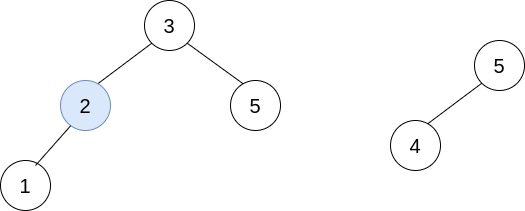# 题目

You are given `n` BST (binary search tree) root nodes for `n` separate BSTs stored in an array `trees` (0-indexed). Each BST in `trees` has at most 3 nodes, and no two roots have the same value. In one operation, you can:

• Select two distinct indices `i` and `j` such that the value stored at one of the leaves of `trees[i]` is equal to the root value of `trees[j]`.
• Replace the leaf node in `trees[i]` with `trees[j]`.
• Remove `trees[j]` from `trees`.

Return the root of the resulting BST if it is possible to form a valid BST after performing `n - 1` operations, or `null` if it is impossible to create a valid BST.

A BST (binary search tree) is a binary tree where each node satisfies the following property:

• Every node in the node’s left subtree has a value strictly less than the node’s value.
• Every node in the node’s right subtree has a value strictly greater than the node’s value.

A leaf is a node that has no children.

Example 1:

```Input: trees = [[2,1],[3,2,5],[5,4]]
Output: [3,2,5,1,null,4]
Explanation:
In the first operation, pick i=1 and j=0, and merge trees into trees.
Delete trees, so trees = [[3,2,5,1],[5,4]].In the second operation, pick i=0 and j=1, and merge trees into trees.
Delete trees, so trees = [[3,2,5,1,null,4]].The resulting tree, shown above, is a valid BST, so return its root.```

Example 2:

```Input: trees = [[5,3,8],[3,2,6]]
Output: []
Explanation:
Pick i=0 and j=1 and merge trees into trees.
Delete trees, so trees = [[5,3,8,2,6]].The resulting tree is shown above. This is the only valid operation that can be performed, but the resulting tree is not a valid BST, so return null.
```

Example 3:

```Input: trees = [[5,4],]
Output: []
Explanation: It is impossible to perform any operations.
```

Constraints:

• `n == trees.length`
• `1 <= n <= 5 * 104`
• The number of nodes in each tree is in the range `[1, 3]`.
• Each node in the input may have children but no grandchildren.
• No two roots of `trees` have the same value.
• All the trees in the input are valid BSTs.
• `1 <= TreeNode.val <= 5 * 104`.

# 分析&代码

Idea
First we build a leaf-value-to-root dict. If we found two leaves with the same value, return None.
Then we iterate the trees, if a tree has same value with other leaf(by checking if tree.val is in the dict), it can be merged into it. Otherwise it’s our root tree!
After we finish merging, check if the tree is a valid bst and node count is right.
Done.

Some Questions

• Should we check if there are circles in the tree?
• technically, yes. However, we have already make sure that won’t happen.
• think these two cases: [[1,null,3],[3,1],[4,2]] and [[1,null,3],[3,1]]
• if circle appears, we will get an incomplete tree after the merging process, node counting will help us check it out.
• if circle appears during the merge, it won’t be the root node, so we don’t need to worry about infinite recursion during node counting

Complexity

Time complexity: O(N)
Space complexity: O(N)

``````# Definition for a binary tree node.
# class TreeNode(object):
#     def __init__(self, val=0, left=None, right=None):
#         self.val = val
#         self.left = left
#         self.right = right
class Solution(object):
def canMerge(self, trees):
"""
:type trees: List[TreeNode]
:rtype: TreeNode
"""

# check if root is a valid bst
# l < root.val < r
def _is_validate(root, l, r):
if root is None:
return True
if root.val <= l or root.val >= r:
return False
if not _is_validate(root.left, l, min(root.val, r)):
return False
if not _is_validate(root.right, max(l, root.val), r):
return False
return True

# calculate node count of root
def _node_cnt(root):
if root is None:
return 0
return 1 + _node_cnt(root.left) + _node_cnt(root.right)

# m : {key : (root, direction)}
# key if val of the leaf node, val is it's root
m = {}
_total_node_cnt = 0
for root in trees:
_total_node_cnt += 1
if root.left:
if root.left.val in m:
# if there are two leaf nodes with the same val, trees cannot merge into one valid bst.
# because leaf values must STRICTLY less or greater than root.
return None
m[root.left.val] = (root, 'l')
_total_node_cnt += 1
if root.right:
if root.right.val in m:
return None
m[root.right.val] = (root, 'r')
_total_node_cnt += 1

ret = None
for root in trees:
if root.val in m:
# root can be merged into other tree
r, dir_ = m[root.val]
if dir_ == 'l':
r.left = root
else:
r.right = root
# merge success, two nodes are merged into one.
_total_node_cnt -= 1
elif ret is not None:
# only one tree("root tree") cannot be merged, return None
return None
else:
# if root cannot merge into other tree, we know it's root of the answer
ret = root

return ret if ret and _node_cnt(ret) == _total_node_cnt and _is_validate(ret, -999999, 999999) else None``````

——此处是内容的分割线——